# Spherical cap + sphere - math problems

#### Number of problems found: 27

• Spherical capFrom the sphere of radius 11 was truncated spherical cap. Its height is 6. What part of the volume is a spherical cap from the whole sphere?
• Spherical cap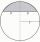What is the surface area of a spherical cap, the base diameter 20 m, height 2 m.
• Spherical capThe spherical cap has a base radius of 8 cm and a height of 5 cm. Calculate the radius of a sphere of which this spherical cap is cut.
• Spherical capPlace a part of the sphere on a 4.6 cm cylinder so that the surface of this section is 20 cm2. Determine the radius r of the sphere from which the spherical cap was cut.
• Sphere - partsCalculate the area of a spherical cap, which is part of an area with base radius ρ = 9 cm and a height v = 3.1 cm.
• Spherical segmentThe spherical segment with height h=1 has a volume V=223. Calculate the radius of the sphere of which is cut this segment.
• Spherical tank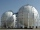The tank of a water tower is a sphere of radius 35ft. If the tank is filled to one quarter of full, what is the height of the water?
• Sphere cutA sphere segment is cut off from a sphere k with radius r = 1. The volume of the sphere inscribed in this segment is equal to 1/6 of the volume of the segment. What is the distance of the cutting plane from the center of the sphere?
• Spherical segmentCalculate the volume of a spherical segment 18 cm high. The diameter of the lower base is 80 cm, the upper base 60 cm.A domed stadium is in the shape of spherical segment with a base radius of 150 m. The dome must contain a volume of 3500000 m³. Determine the height of the dome at its centre to the nearest tenth of a meter.
• Hemisphere cutCalculate the volume of the spherical layer that remains from the hemisphere after the 3 cm section is cut. The height of the hemisphere is 10 cm.
• Sphere parts, segmentA sphere with a diameter of 20.6 cm, the cut is a circle with a diameter of 16.2 cm. .What are the volume of the segment and the surface of the segment?
• The roof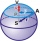The roof has the shape of a spherical canopy with a base diameter of 8 m and a height of 2 m, calculate the area of the foil with which the roof is covered, when we calculate 13% for waste and residues.
• PilotHow high is the airplane's pilot to see 0.001 of Earth's surface?
• Elevation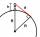What must be the elevation of an observer in order that he may be able to see an object on the earth 536 km away? Assume the earth to be a smooth sphere with radius 6378.1 km.
• AirplaneAviator sees part of the earth's surface with an area of 200,000 square kilometers. How high he flies?
• Float boya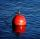A 0.5 meter spherical float is used as a location mark for a fishing boat anchor. It floats in salt water. Find the depth to which the float sinks if the material of which the float is made weighs 8 kilograms per cubic meter and salt water weighs 1027 kg/
• Felix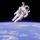Calculate how much land saw Felix Baumgartner after jump from 32 km above ground. The radius of the Earth is R = 6378 km.
• Above EarthTo what height must a boy be raised above the earth in order to see one-fifth of its surface.
• The hemisphereThe hemisphere container is filled with water. What is the radius of the container when 10 liters of water pour from it when tilted 30 degrees?

Do you have an interesting mathematical word problem that you can't solve it? Submit a math problem, and we can try to solve it.

We will send a solution to your e-mail address. Solved examples are also published here. Please enter the e-mail correctly and check whether you don't have a full mailbox.

Please do not submit problems from current active competitions such as Mathematical Olympiad, correspondence seminars etc...

Spherical cap Problems. Sphere Problems.